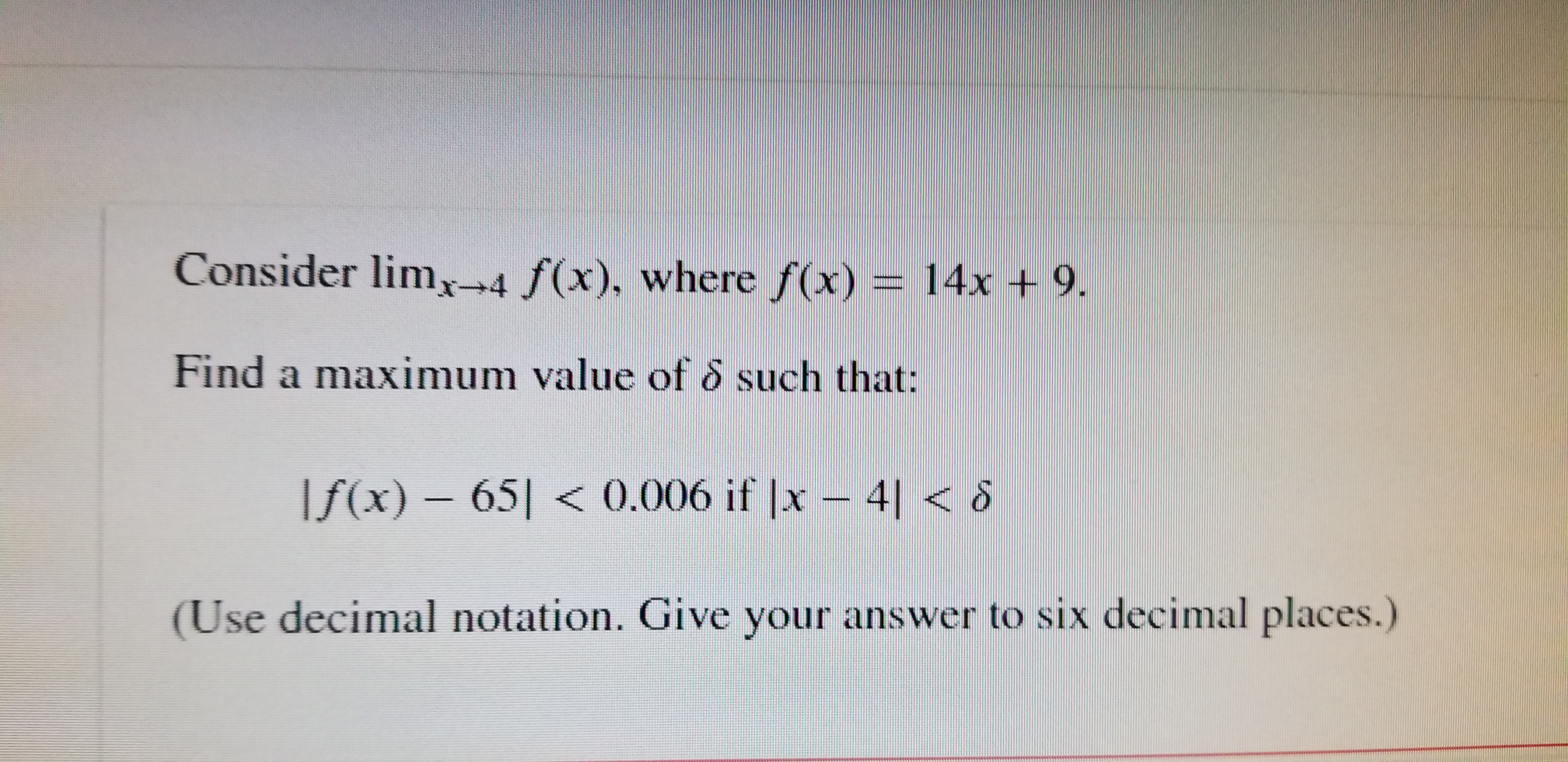# Consider limx-4 f(x), where f(x) 14x+ 9. Find a maximum value of 6 such that: If(x) 65< 0.006 if |x 41< (Use decimal notation. Give your answer to six decimal places.)

Questionhelp_outlineImage TranscriptioncloseConsider limx-4 f(x), where f(x) 14x+ 9. Find a maximum value of 6 such that: If(x) 65< 0.006 if |x 41< (Use decimal notation. Give your answer to six decimal places.) fullscreen

### Want to see this answer and more?

Experts are waiting 24/7 to provide step-by-step solutions in as fast as 30 minutes!*

*Response times vary by subject and question complexity. Median response time is 34 minutes and may be longer for new subjects.
Tagged in
MathCalculus

### Other